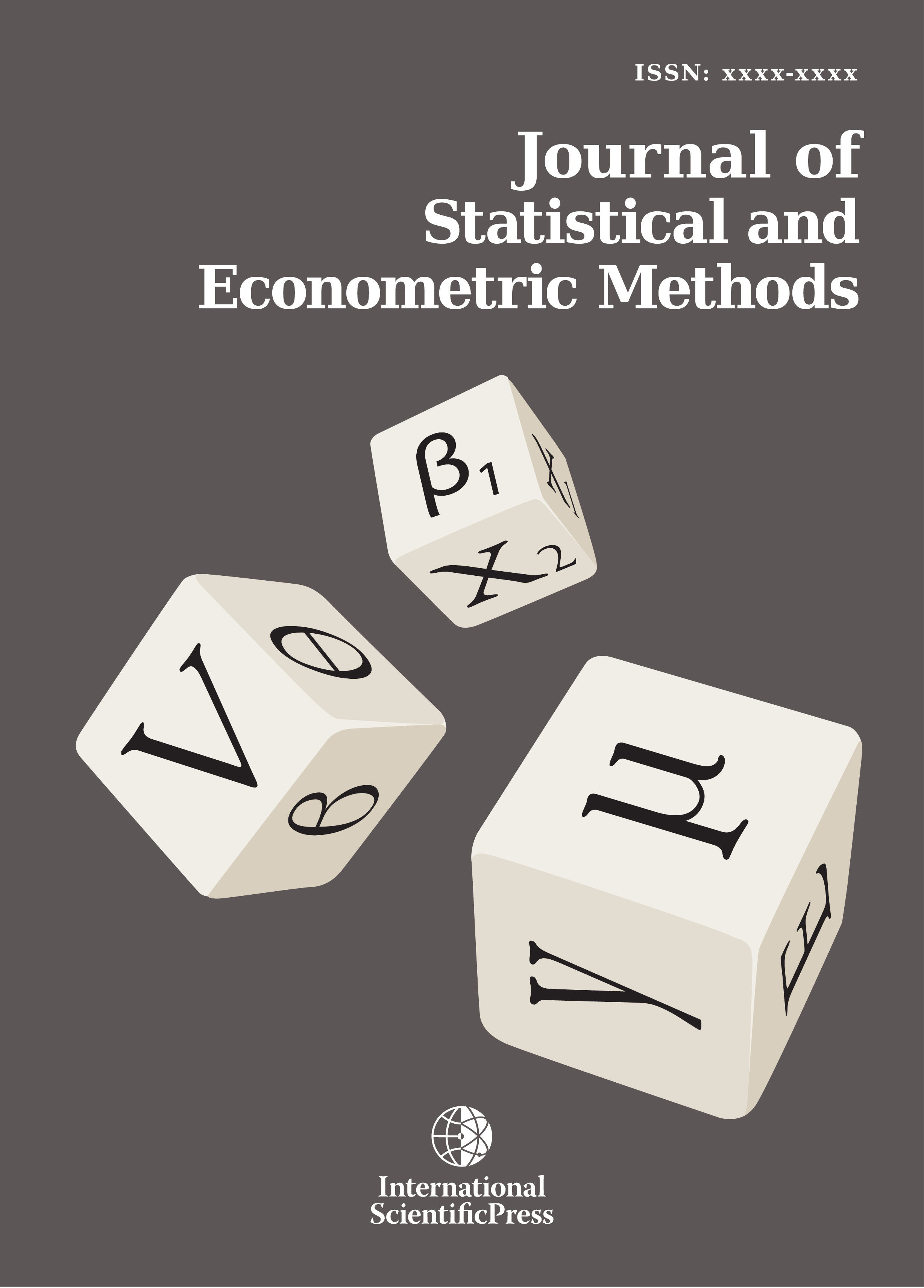# Journal of Statistical and Econometric Methods

#### Ruin Probability in a generalized risk process out interest force with homogenous Markov chain premiums

•[ Download ]
• Times downloaded: 1371
• Abstract

In this paper, we consider generalized risk processes out interest force with assumption that sequence of premiums is homogenous markov chain, takes a finite number of possible integer values and claims are independent and identically distributed non – negative random variables with the same distributive function. The state space of premiums in this paper is finite, which it satisfies with cases of practice. The aim of this paper is to give recursive equations for finite time ruin probabilities and integral equation for ultimate ruin probability of generalized risk processes out interest force with homogenous markov chain premiums and it establish Generalized Lundberg inequalities for ruin probabilities. Generalized Lundberg inequalities for ruin probabilities are derived by using recursive technique. Theorem 2.1 give recursive equations for finite time ruin probabilities and integral equation for ultimate ruin probability. To establish probability inequalities for finite time ruin probabilities and ultimate ruin probability of this model, we built Lemma 3.1 to define a adjustment coefficient R0>0, this coefficient is belong to initial value of premiums. Using by Theorem 2.1 and Lemma 3.1, we establish Theorem 3.1, which it give probability inequality for ultimate ruin probability by an inductive approach. Exponential upper bounds for the finite time ruin probabilities and ultimate ruin probability were obtained in Theorem 3.1.ISSN: 2241-0376 (Online)
2241-0384 (Print)